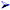#Five Beginner and Intermediate Futoshiki Solving StrategiesIf you are a Futoshiki player, then you know that it can be quite a challenging type of puzzle if not harder than Sudoku and different from Kakuro. The objective is to place numbers in the white squares (called cells) so that each row and column contain each of the digits only once. No duplicate digits are allowed in any row or column. Also, you will only use digits based on the size of the grid. So for example, if you are playing a 5 x 5 grid, only digits 1 through 5 will be used. For a 8 x 8 grid, only 1 through 8 is used.

There are a few key rules in playing:In a N x N puzzle the range of #'s must be between 1 and N.No digit is repeated within row or column. No duplicates.Inequality constraints must be honored: 5 > 4, 5 > 3, or 1 < 4Like sudoku, there are many techniques in solving a futoshiki puzzle. When the simple techniques don't work, you will need to use a more complicated solving technique. This can get to a point where it almost looks impossible to solve and may take a long time using a variety of strategies. But keep in mind, just like Sudoku and Kakuro, a Futoshiki puzzle has only one unique solution that will work successfully. In this article I will give you a few futoshiki strategies.

Strategy #1: Use Pencil marks

This is by far the first (and in my opinion most important) strategy, using pencil marks! Using pencil marks not only greatly reduce the chance for error, it helps to point out obvious hints in solving puzzles. Now, it seems like I am stating the obvious but it very easy to overlook it and think "I got it, it is no big deal" only to run into a problem later on. Using pencil marks, it is best to fill in possible values that you know are going to exist in a particular box. You don't need to over do it sometimes but it is good for a small range. Then, as more clues are revealed, you can reduce the pencil marks until only one digit remain and that will be the digit used for that box.

Strategy #2: Look for chains or a groups of chains

A good place to start a futoshiki puzzle is to look for chains. A chain is several connected boxes that are linked by the same "greater than" or "less than" sign. They don't necessarily need to be in any specific order just as long they are pointing in a specific direction. In a 6x6 puzzle Example A you can see a chain and thus you now know definitely what the values af the boxes are. In a 7x7 puzzle Example B you can see a chain but don't know exactly what values they are but you can deduce that it is a range of values.

 Example A (6x6)Example B (6x6)Strategy #3: Pairs, Triples, Quadruples

This is a very good strategy for sudoku, kakuro and futoshiki puzzles assuming you are using pencil marks. If a box of a given row (or column) only has a pencil mark of 1 and 5 and a box in the same row (or column) also has a pencil mark of 1 and 5 then you can remove 1 and 5 from all other boxes in that row (or column). The reason behind this is that the first box must be a 1 or a 5 and the second box must be a 1 or a 5. If the 3rd box was set as a 5 for example, it would cause an obvious error in the row since you are not allowed to have the same digit in a row or column.

 Example Pair (5x5 puzzle)Example Triple (6x6 puzzle)Strategy #4: Lows and Highs

The unique thing about a futoshiki puzzle is that it is based off of inqeuality signs. This opens up the door to look for the high and low numbers to start off a puzzle. You can do this by looking for only one box in any given row or column that has all inequality signs either pointing in (for low) or pointing out (for high). Then you can determine exactly what that number is without any further guesswork. In the Example Low you can only put a 1 here because anywhere else if you put a 1 a "greater than" sign would mean that the adjacent box would be a zero. In the Example High you can only put a 6 here because anywhere else if you put a 6 a "less than" sign would mean that the adjacent box would be a seven and that is invalid in a 6x6 puzzle.

 Example Low (6x6 puzzle)Ā
 Example High (6x6 puzzle)Keep in mind that this strategy will only work if only one box in a row or column looks like this. If more than one row or column has this than there is more possibilities that either one could be a 1 or 6.

Strategy #5: Testing Digits (or Pencil-marks)

Here is one more final strategy for you which can be quite useful. It is using the "what if this box was a specific #?" scenario -or- "I know this box is a 1,4, or 5 so if I put a 4 here would I run into a snag?" From there, you can see if other digits will work out. If you find a snag in the end then you can eliminate that specific possibility (or pencil-mark) from that box to begin with.

Good luck on your next Futoshiki puzzle!